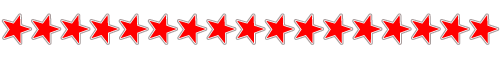Like   Tweet   Pin   +1   intable div table+table div table{width:100%;padding:0}table div table+table div table table{padding:0;float:left!important;width:51.886%!important}table div table+table div table table+table td,table div table+table div table td{padding-left:0;padding-right:0}table div table+table div table table td{padding-left:0;padding-right:20px}table div table+table div table table+table{float:left!important;width:48.114%!important}/* styles */
 table div table+table+table div table{width:100%;padding:0}table div table+table+table div table table{padding:0;float:left!important;width:51.886%!important}table div table+table+table div table table+table td,table div table+table+table div table td{padding-left:0;padding-right:0}table div table+table+table div table table td{padding-left:0;padding-right:20px}table div table+table+table div table table+table{float:left!important;width:48.114%!important}/* styles */
 table div table+table+table+table div table{width:100%;padding:0}table div table+table+table+table div table table{padding:0;float:left!important;width:51.886%!important}table div table+table+table+table div table table+table td,table div table+table+table+table div table td{padding-left:0;padding-right:0}table div table+table+table+table div table table td{padding-left:0;padding-right:20px}table div table+table+table+table div table table+table{float:left!important;width:48.114%!important}/* styles */
 table div table+table+table+table+table div table{width:100%;padding:0}table div table+table+table+table+table div table table{padding:0;float:left!important;width:51.886%!important}table div table+table+table+table+table div table table+table td,table div table+table+table+table+table div table td{padding-left:0;padding-right:0}table div table+table+table+table+table div table table td{padding-left:0;padding-right:20px}table div table+table+table+table+table div table table+table{float:left!important;width:48.114%!important}/* styles */
 table div table+table+table+table+table+table div table td,table.module-5{width:100%;padding:0}table div table+table+table+table+table+table div table{width:100%;float:none;margin-left:auto;margin-right:auto;padding:0}table div table+table+table+table+table+table div table a{border:0 none;text-decoration:none}table div table+table+table+table+table+table div table img{width:100%!important;border:0 none;text-decoration:none}/* styles */
 table div table+table+table+table+table+table+table div table{width:100%;padding:0}table div table+table+table+table+table+table+table div table table{padding:0;float:left!important;width:51.886%!important}table div table+table+table+table+table+table+table div table table+table td,table div table+table+table+table+table+table+table div table td{padding-left:0;padding-right:0}table div table+table+table+table+table+table+table div table table td{padding-left:0;padding-right:20px}table div table+table+table+table+table+table+table div table table+table{float:left!important;width:48.114%!important}/* styles */
 table div table+table+table+table+table+table+table+table div table{width:100%;padding:0}table div table+table+table+table+table+table+table+table div table table{padding:0;float:left!important;width:51.886%!important}table div table+table+table+table+table+table+table+table div table table+table td,table div table+table+table+table+table+table+table+table div table td{padding-left:0;padding-right:0}table div table+table+table+table+table+table+table+table div table table td{padding-left:0;padding-right:20px}table div table+table+table+table+table+table+table+table div table table+table{float:left!important;width:48.114%!important}/* styles */
 table div table+table+table+table+table+table+table+table+table div table{width:100%;padding:0}table div table+table+table+table+table+table+table+table+table div table table{padding:0;float:left!important;width:51.886%!important}table div table+table+table+table+table+table+table+table+table div table table+table td,table div table+table+table+table+table+table+table+table+table div table td{padding-left:0;padding-right:0}table div table+table+table+table+table+table+table+table+table div table table td{padding-left:0;padding-right:20px}table div table+table+table+table+table+table+table+table+table div table table+table{float:left!important;width:48.114%!important}/* styles */
 table div table+table+table+table+table+table+table+table+table+table div table{width:100%;padding:0}table div table+table+table+table+table+table+table+table+table+table div table table{padding:0;float:left!important;width:51.886%!important}table div table+table+table+table+table+table+table+table+table+table div table table+table td,table div table+table+table+table+table+table+table+table+table+table div table td{padding-left:0;padding-right:0}table div table+table+table+table+table+table+table+table+table+table div table table td{padding-left:0;padding-right:20px}table div table+table+table+table+table+table+table+table+table+table div table table+table{float:left!important;width:48.114%!important}/* styles */table div table+table+table+table+table+table+table+table+table+table+table+table div table td,table.module-11{width:100%;padding:0}table div table+table+table+table+table+table+table+table+table+table+table+table div table{width:100%;float:none;margin-left:auto;margin-right:auto;padding:0}table div table+table+table+table+table+table+table+table+table+table+table+table div table a{border:0 none;text-decoration:none}table div table+table+table+table+table+table+table+table+table+table+table+table div table img{width:100%!important;border:0 none;text-decoration:none}/* styles */Reach out to promoters and beyond with your message, photos and videos via Raestar's Promotions | Danielle White +44 (0)7841 675 263 | daniraestar@aol.com | www.daniraestar.com #BLACKLIVESMATTER
JAZZ ALBUM SHOWCASE
LATEST FEATURE E-BLAST